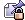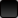Author Topic: ScriptBasic Keyword List  (Read 1767 times)

John Spikowski

• Moderator
••• Posts: 224ScriptBasic Keyword List
« on: April 18, 2019, 02:42:02 am »
This is the ScriptBasic keyword list.

ABS
ACOS
ACOSECANT
ACTAN
ALIAS
AND
AS
ASC
ASECANT
ASIN
ATAN
ATN
BIN
BINMODE
BY
BYVAL
CALL
CHDIR
CHOMP
CHR
CHR\$
CINT
CLOSE
CLOSEALL
COMMAND
CONF
CONST
COS
COSECANT
COTAN
COTAN2
CRYPT
CURDIR
CVD
CVI
CVL
CVS
DAY
DECLARE
DELETE
DELTREE
DIRECTORY
DO
ELIF
ELSE
ELSEIF
ELSIF
END
ENDIF
ENVIRON
ENVIRON\$
EOD
EOF
ERROR
ERROR\$
EVEN
EXECUTE
EXIT
EXP
FALSE
FILE
FILEACCESSTIME
FILECOPY
FILECREATETIME
FILEEXISTS
FILELEN
FILEMODIFYTIME
FILEOWNER
FIX
FOR
FORK
FORMAT
FORMATDATE
FORMATTIME
FRAC
FREEFILE
FROM
FUNCTION
GCD
GLOBAL
GMTIME
GMTIMETOLOCALTIME
GO
GOSUB
GOTO
HCOS
HCOSECANT
HCTAN
HEX
HEX\$
HOSTNAME
HOUR
HSECANT
HSIN
HTAN
ICALL
IF
IMAX
IMIN
INPUT
INSTR
INSTRREV
INT
ISARRAY
ISDEFINED
ISDIRECTORY
ISEMPTY
ISFILE
ISINTEGER
ISNUMERIC
ISREAL
ISSTRING
ISUNDEF
JOIN
JOKER
KILL
LBOUND
LCASE
LCASE\$
LCM
LEFT
LEFT\$
LEN
LET
LIB
LIKE
LINE
LOC
LOCAL
LOCALTIMETOGMTIME
LOCK
LOF
LOG
LOG10
LOOP
LOWER
LOWER\$
LTRIM
LTRIM\$
MAX
MAXINT
MID
MID\$
MIN
MININT
MINUTE
MKD
MKD\$
MKDIR
MKI
MKI\$
MKL
MKL\$
MKS
MKS\$
MODULE
MONTH
NAME
NEXT
NEXTFILE
NO
NOT
NOW
NULL
OCT
OCT\$
ODD
ON
OPEN
OPTION
OR
OUTPUT
PACK
PATTERN
PAUSE
PI
POP
POW
PRINT
PRINTNL
QUOTE
RANDOMIZE
REF
REGION
REPEAT
REPLACE
RESET
RESUME
RETURN
REWIND
RIGHT
RIGHT\$
RND
ROUND
RTRIM
RTRIM\$
SEC
SECANT
SEEK
SET
SGN
SIN
SLEEP
SPACE
SPACE\$
SPLIT
SPLITA
SPLITAQ
SQR
STEP
STOP
STR
STR\$
STRING
STRING\$
STRREVERSE
STRREVERSE\$
SUB
SWAP
SYSTEM
TAN
TAN2
TEXTMODE
THEN
TIME
TIMEVALUE
TO
TRIM
TRIM\$
TRUE
TRUNCATE
TYPE
UBOUND
UCASE
UCASE\$
UNDEF
UNPACK
UNTIL
UPPER
UPPER\$
VAL
VAR
WAITPID
WEEKDAY
WEND
WHILE
WILD
XOR
YEAR
YEARDAY

Not Implemented
• BIN - This is a planned function to convert the argument number to binary format. (aka. format as a binary number containing only 0 and 1 characters and return this string)
• GCD - Mathematical function has become a reserved word, but are not implemented.
• LCM - Mathematical function has become a reserved word, but are not implemented.
• ATN - This is a planned function to calculate the arcus tangent of the argument.
• ATAN - This is a planned function to calculate the arcus tangent of the argument.
• TAN - This is a planned function to calculate the tangent of the argument.
• TAN2 - This is a planned function to calculate the tangent of the ratio of the two arguments.
• COTAN - This is a planned function to calculate the cotangent of the argument.
• COTAN2 - This is a planned function to calculate the cotangent of the ratio of the two arguments.
• ACTAN - This is a planned function to calculate the arcus cotangent of the argument.
• SECANT - This is a planned function to calculate the secant of the argument.
• COSECANT - This is a planned function to calculate the cosecant of the argument.
• ASECANT - This is a planned function to calculate the arcus secant of the argument.
• ACOSECANT - This is a planned function to calculate the arcus cosecant of the argument.
• HSIN - This is a planned function to calculate the sinus hyperbolicus of the argument.
• HCOS - This is a planned function to calculate the cosinus hyperbolicus of the argument.
• HTAN - This is a planned function to calculate the tangent hyperbolicus of the argument.
• HCTAN - This is a planned function to calculate the cotangent hyperbolicus of the argument.
• HSECANT - This is a planned function to calculate the secant hyperbolicus of the argument.
• HCOSECANT - This is a planned function to calculate the cosecant hyperbolicus of the argument.
• MAX - This is a planned function to select and return the maximum of the arguments.
• MIN - This is a planned function to select and return the minimum of the arguments.
• IMAX - This is a planned function to select and return the index of the maximum of the arguments.
• IMIN - This is a planned function to select and return the index of the minimum of the arguments.
• CVD - This is a planned function to convert the argument string into a real number. (8 byte)
• CVI - This is a planned function to convert the argument string into an integer. (2 bytes)
• CVL - This is a planned function to convert the argument string into an long integer. (4 bytes)
• CVS - This is a planned function to convert the argument string into an integer. (4 byte)
• MKD - This is a planned function to convert the argument real number to an 8 byte string. (8 byte)
• MKI - This is a planned function to convert the argument integer number to a string. (2 byte)
• MKS - This is a planned function to converts a single-precision number "n" into a  string so it can later be retrieved from a random-access file as a numeric value. (4 byte)
• MKL - This is a planned function converts a long-integer number "n" into a string so it can later be retrieved from a random-access file as a numeric value. (4-byte)

Valid ScriptBasic Operators

Power operator (^)
Multiplication operator (*)
Division operator (/)
Integer division operator (\)
Modulus operator (%)
Addition and subtraction operators (+, -)
Bit-wise and logical NOT (NOT)
Equality operator (=)
Not equal operator (<>)
Compare operators (<, <=, >, >=)
Logical operators (and, or, xor)
Concatenation operator (&)
ByVal operator
LIKE operator

Operators that are undefined by default but can be used by external modules are:

?     !     #     `     @
+^    +<    +>    +?    +=    +*    +/    +%    +!    +#    +&    +\    +`    +'
+@    -^    -<    ->    -?    -=    -*    -/    -%    -!    -#    -&    -\    -`
-'    -@    ^^    ^<    ^>    ^?    ^=    ^*    ^/    ^%    ^!    ^#    ^&    ^\
^`    ^'    ^@    <^    <<    <?    <*    </    <%    <!    <#    <&    <\    <`
<'    <@    >^    ><    >>    >?    >*    >/    >%    >!    >#    >&    >\    >`
>'    >@    ?^    ?<    ?>    ??    ?=    ?*    ?/    ?%    ?!    ?#    ?&    ?\
?`    ?'    ?@    =^    =<    =>    =?    ==    =*    =/    =%    =!    =#    =&
=\    =`    ='    =@    *^    *<    *>    *?    *=    **    */    *%    *!    *#
*&    *\    *`    *'    *@    /^    /<    />    /?    /=    /*    //    /%    /!
/#    /&    /\    /`    /'    /@    %^    %<    %>    %?    %=    %*    %/    %%
%!    %#    %&    %\    %`    %'    %@    !^    !<    !>    !?    !=    !*    !/
!%    !!    !#    !&    !\    !`    !'    !@    #^    #<    #>    #?    #=    #*
#/    #%    #!    ##    #&    #\    #`    #'    #@    &^    &<    &>    &?    &=
&*    &/    &%    &!    &#    &&    &\    &`    &'    &@    \^    \<    \>    \?

\=    \*    \/    \%    \!    \#    \&    \\    \`    \'    \@    `^    `<    `>
`?    `=    `*    `/    `%    `!    `#    `&    `\    ``    `'    `@    '^    '<
'>    '?    '=    '*    '/    '%    '!    '#    '&    '\    '`    ''    '@    @^
@<    @>    @?    @=    @*    @/    @%    @!    @#    @&    @\    @`    @'    @@

« Last Edit: April 18, 2019, 03:06:39 am by admin »
ScriptBasic Project Manager/Facilitator